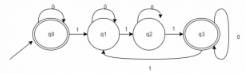Test: Applications of DFA

# Test: Applications of DFA - Computer Science Engineering (CSE)

Test Description

## 10 Questions MCQ Test Theory of Computation - Test: Applications of DFA

Test: Applications of DFA for Computer Science Engineering (CSE) 2023 is part of Theory of Computation preparation. The Test: Applications of DFA questions and answers have been prepared according to the Computer Science Engineering (CSE) exam syllabus.The Test: Applications of DFA MCQs are made for Computer Science Engineering (CSE) 2023 Exam. Find important definitions, questions, notes, meanings, examples, exercises, MCQs and online tests for Test: Applications of DFA below.
Solutions of Test: Applications of DFA questions in English are available as part of our Theory of Computation for Computer Science Engineering (CSE) & Test: Applications of DFA solutions in Hindi for Theory of Computation course. Download more important topics, notes, lectures and mock test series for Computer Science Engineering (CSE) Exam by signing up for free. Attempt Test: Applications of DFA | 10 questions in 10 minutes | Mock test for Computer Science Engineering (CSE) preparation | Free important questions MCQ to study Theory of Computation for Computer Science Engineering (CSE) Exam | Download free PDF with solutions
 1 Crore+ students have signed up on EduRev. Have you?
Test: Applications of DFA - Question 1

### Given Language: {x | it is divisible by 3} The total number of final states to be assumed in order to pass the number constituting {0, 1} is

Detailed Solution for Test: Applications of DFA - Question 1

The DFA for the given language can be constructed as follows:Test: Applications of DFA - Question 2

### A binary string is divisible by 4 if and only if it ends with:

Detailed Solution for Test: Applications of DFA - Question 2

If the string is divisible by four, it surely ends with the substring ‘100’ while a binary string divisible by 2 would surely end with the substring ‘10’.

Test: Applications of DFA - Question 3

### Let L be a language whose FA consist of 5 acceptance states and 11 non final states. It further consists of a dumping state. Predict the number of acceptance states in Lc.

Detailed Solution for Test: Applications of DFA - Question 3

If L leads to FA1, then for Lc, the FA can be obtained by exchanging the final and non-final states.

Test: Applications of DFA - Question 4

If L1 and L2 are regular languages, which among the following is an exception?

Detailed Solution for Test: Applications of DFA - Question 4

It the closure property of Regular language which lays down the following statement:
If L1, L2 are 2- regular languages, then L1 U L2, L1 ∩ L2, L1C, L1 – L2 are regular language.

Test: Applications of DFA - Question 5

Predict the analogous operation for the given language:
A: {[p, q] | p ϵ A1, q does not belong to A2}

Detailed Solution for Test: Applications of DFA - Question 5

When set operation ‘-‘ is performed between two sets, it points to those values of prior set which belongs to it but not to the latter set analogous to basic subtraction operation.

Test: Applications of DFA - Question 6

Which among the following NFA’s is correct corresponding to the given Language?
L= {xϵ {0, 1} | 3rd bit from right is 0}

Detailed Solution for Test: Applications of DFA - Question 6

The NFA accepts all binary strings such that the third bit from right end is 1 and if not, is send to Dumping state. Note: It is assumed that the input is given from the right end bit by bit.

Test: Applications of DFA - Question 7

Statement 1: NFA computes the string along parallel paths.Statement 2: An input can be accepted at more than one place in an NFA.Which among the following options are most appropriate?

Detailed Solution for Test: Applications of DFA - Question 7

While the machine runs on some input string, if it has the choice to split, it goes in all possible way and each one is different copy of the machine. The machine takes subsequent choice to split further giving rise to more copies of the machine getting each copy run parallel. If any one copy of the machine accepts the strings, then NFA accepts, otherwise it rejects.

Test: Applications of DFA - Question 8

Which of the following options is correct for the given statement?
Statement: If K is the number of states in NFA, the DFA simulating the same language would have states less than 2k.

Detailed Solution for Test: Applications of DFA - Question 8

If K is the number of states in NFA, the DFA simulating the same language would have states equal to or less than 2k.

Test: Applications of DFA - Question 9

Let N (Q, ∑, δ, q0, A) be the NFA recognizing a language L. Then for a DFA (Q’, ∑, δ’, q0’, A’), which among the following is true?

Detailed Solution for Test: Applications of DFA - Question 9

All the optioned mentioned are the instruction formats of how to convert a NFA to a DFA.

Test: Applications of DFA - Question 10

There exists an initial state, 17 transition states, 7 final states and one dumping state, Predict the maximum number of states in its equivalent DFA?

Detailed Solution for Test: Applications of DFA - Question 10

The maximum number of states an equivalent DFA can comprise for its respective NFA with k states will be 2k.

## Theory of Computation

18 videos|56 docs|44 tests
Information about Test: Applications of DFA Page
In this test you can find the Exam questions for Test: Applications of DFA solved & explained in the simplest way possible. Besides giving Questions and answers for Test: Applications of DFA, EduRev gives you an ample number of Online tests for practice

## Theory of Computation

18 videos|56 docs|44 tests